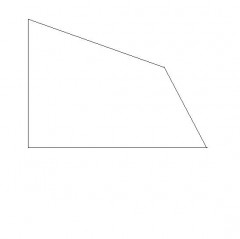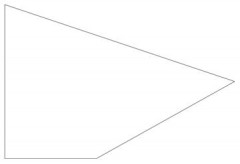# How To Draw A Quadrangle With Only One Right Angle?

A quadrangle, also known as a quadrilateral, is a geometrical shape that consists of four straight sides and four corners.

The only condition that restricts the drawing of a quadrangle is that all four of the corners must equate to 360 degrees.
It is also possible to draw quadrangles with at least one right angle. Below are some instructions on how to do this and the equipment that you will need to complete the task.
You will need:
· Paper.
· Ruler.
· Pencil.
· Protractor.
· Rubber (if a mistake is made).

Instructions:
· The first thing you will need to do is to draw a straight line on the piece of paper using the ruler and a pencil. This can be any length you like.
· Using your pencil, mark a point anywhere on the line you have just drawn and use the protractor to draw 90 degrees.
· Line up the baseline of the protractor with the straight pencil line, making sure that the middle of the protractor is directly over the pencil point you marked out.
· Using the protractor, carefully measure a 90 degree angle away from the straight pencil line and accurately mark it.
· Using the ruler and pencil, join the pencil mark on the straight line to the 90 degree pencil mark. This will form a right angle.
· Use the ruler and pencil to draw a third straight line on the opposite side to the right angle. You can draw this line in any direction.
· Finish your quadrangle by joining up the end of the third line to the end of the first one. Now you will have a quadrangle or quadrilateral that contains one right angle.
Once you have finished, use your protractor to make sure all the angles add up to 360 degrees.
thanked the writer.thanked the writer.
Here is one.thanked the writer.
A kite
thanked the writer.
A kite
thanked the writer.
A kite
thanked the writer.
A trapezoid
thanked the writer.